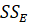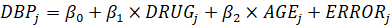ANCOVA

Example Codes:

Analysis of Covariance (ANCOVA) is a blend of ANOVA that analyzes the difference of means of a continuous response variable across levels of a categorical predictor, controlling for the effect of covariates that are of less interest.

ANCOVA can explain the within-group error variance and hence reduce it. It tries to explain the unexplained variance () in ANOVA in terms of covariates. Additionally, ANCOVA helps to eliminate the influence on results by confounders. The power of the ANCOVA test would increase when entering a strong covariate and be reduced by having a weak covariate.

Assumptions

For the ANCOVA test, the predictor is categorical, while the response variable and covariates are continuous.

There are five assumptions of ANCOVA:

- Linearity: the covariates and the response variable are linearly related.

- Homogeneity of variance: the error is a random variable with zero mean and equal variance across all subpopulations.

- Homogeneity of regression slopes: the slopes of regression lines are equal across all subpopulations.

- Independence: observations are independent, which means errors are uncorrelated.

- Normality: The errors follow a normal distribution.

Model

Suppose a researcher is studying the relationship between the decrease in a person’s blood pressure (DBP) and personal and environmental variables, to which they are exposed. Knowledge from observational studies and laboratory findings suggest that the decrease in a person’s blood pressure is related to their age and a drug believed to reduce blood pressure.

Then the model can be written asThe main effects of drug treatment on the decrease of blood pressure are tested, controlling for the effect of age.

Example Code in SAS

/* Analysis of Covariance --------------------------------------*/

data DrugTest;

input Drug \$ PreTreatment PostTreatment @@;

datalines;

11  6   A  8  0   A  5  2   A 14  8   A 19 11

A  6  4   A 10 13   A  6  1   A 11  8   A  3  0

D  6  0   D  6  2   D  7  3   D  8  1   D 18 18

D  8  4   D 19 14   D  8  9   D  5  1   D 15  9

F 16 13   F 13 10   F 11 18   F  9  5   F 21 23

F 16 12   F 12  5   F 12 16   F  7  1   F 12 20

;

proc glm data=DrugTest;

class Drug;

model PostTreatment = Drug PreTreatment / solution;

lsmeans Drug / stderr pdiff cov out=adjmeans;

run;

run;

/* Visualize the Fitted Analysis of Covariance Model -----------*/

ods graphics on;

proc glm data=DrugTest plot=meanplot(cl);

class Drug;

model PostTreatment = Drug PreTreatment;

lsmeans Drug / pdiff;

run;

ods graphics off;

Example Code in R

install.packages("jmv")

# load library jmv for function "ancova"

library(jmv)

# input data

drug <- c("A", "A", "A", "A", "A", "A", "A", "A", "A", "A",

"D", "D", "D", "D", "D", "D", "D", "D", "D", "D",

"F", "F", "F", "F", "F", "F", "F", "F", "F", "F")

pre_treatment <- c(11, 8, 5, 14, 19, 6, 10, 6, 11, 3,

6, 6, 7, 8, 18, 8, 19, 8, 5, 15,

16, 13, 11, 9, 21, 16, 12, 12, 7, 12)

post_treatment <- c(6, 0, 2, 8, 11, 4, 13, 1, 8, 0,

0, 2, 3, 1, 18, 4, 14, 9, 1, 9,

13, 10, 18, 5, 23, 12, 5, 16, 1, 20)

# identify drug as a categorical variable

drug <- factor(drug)

# create a data frame

df <- data.frame(drug, pre_treatment, post_treatment)

# ANCOVA

ancova(formula = post_treatment ~ drug + pre_treatment, data=df)

References

1.     Stephanie Glen. "ANCOVA: Analysis of Covariance" From StatisticsHowTo.com: Elementary Statistics for the rest of us! https://www.statisticshowto.com/ancova/

2.     Keppel, G. (1991). Design and analysis: A researcher's handbook (3rd ed.). Englewood Cliffs: Prentice-Hall, Inc.

3.     Tabachnick, B. G.; Fidell, L. S. (2007). Using Multivariate Statistics (5th ed.). Boston: Pearson Education.

4.     Example 48.4 Analysis of Covariance. (n.d.). SAS® Help Center. Retrieved November 3, 2021, from https://documentation.sas.com/doc/en/pgmsascdc/9.4_3.3/statug/statug_glm_examples04.htm